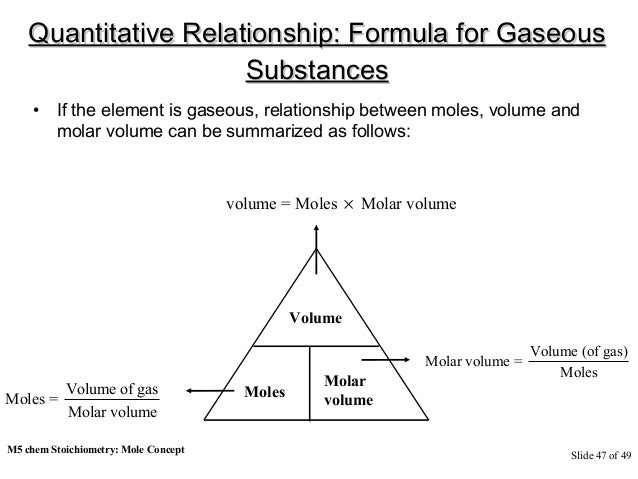# What is the relationship between theory and practice?

There is often a false narrative peddled to the effect that education is only effective if all lessons learnt are administered in practice as opposed to in theory. Indeed, such a narrative has been repeated so often that many students often look at theoretical classes with a degree of contempt. And this always leaves so many questions begging for answers; is there a relationship between theory and practice?

What subjects or courses demand more of one than the other? And are these two principles of learning mutually exclusive?

In this post, we shall go in-depth on the relationship between the two, in a bid to enlightening you on which is more ideal and under what circumstance.

## When Is Theory Ideal?

Nearly everything in life is learnt in theory before it can be put in practice. This, therefore, means that theory is ideal in nearly all courses. It is through theoretical learning that a student is first introduced to a topic. Theoretical aspects of teaching enable a student to develop mental pictures, perhaps even enact scenarios in their minds as well as solve mental problems.

Theoretical lessons are meant to evoke a sense of eagerness on the learner’s mind and enable them ask some pertinent questions. Through theoretical learning, students will be able to pose questions, make comments, develop hypotheses and even contribute towards the furtherance of the theory.

As expected, there are various subjects that are best delivered in theory, an example being religious studies and various humanities subjects. Also, as already been mentioned, most practical subjects are first introduced as theories before they can be proved in real-life demonstrations. For instance, a student will first need to understand the Mole concept and its formula such as:It is only after mastering the formula can the student proceed to a real laboratory titration experiment.

## When Is Practice Ideal?

First off, we will begin by challenging you to dispel the notion that practice is only meant for doubting Thomases. There is a general perception that the main objective of every practical lesson is to prove a theory right or wrong. While there is some truth to that, practice can be looked at as the ideal way of corroborating theories. Through practical lessons, learners are able to take the idea out of their minds and put them into real experiments.

There are topics that a learner cannot fully internalize unless the topic is illustrated in a real-life situation [or a simulation of the same]. For instance, a biology student will need to go on a field excursion so as to understand exactly what a particular tree species looks like. Most notably, practical learning works best for students that are hearing-impaired, as is shown in this video – https://www.youtube.com/watch?v=o1EAoaEIoYQ

## So, What’s The Relationship?

Theory and practice are not mutually exclusive, neither is one more important than the other. A theory is meant to first introduce a new topic or concept while practice proves that the lessons and arguments advanced in the theory are actually true.

While there are lessons that must only be dispensed in theory or practice, there is no denying the fact that the two concepts are interdependent. Therefore, as far as possible, lecturers and tutors must ensure they put their students through the two levels of learning. This way, they can be sure they cater to the needs of all and sundry.